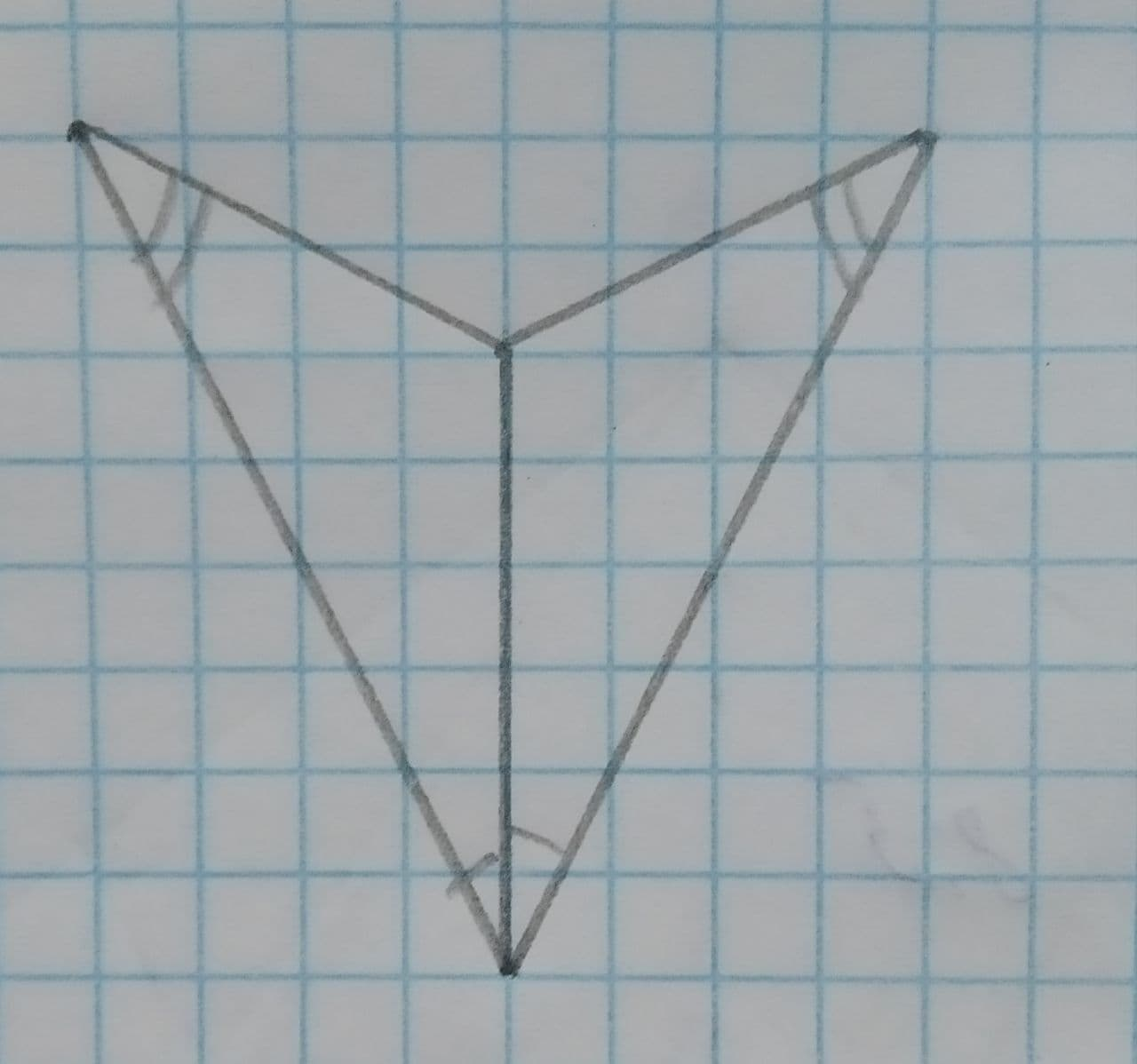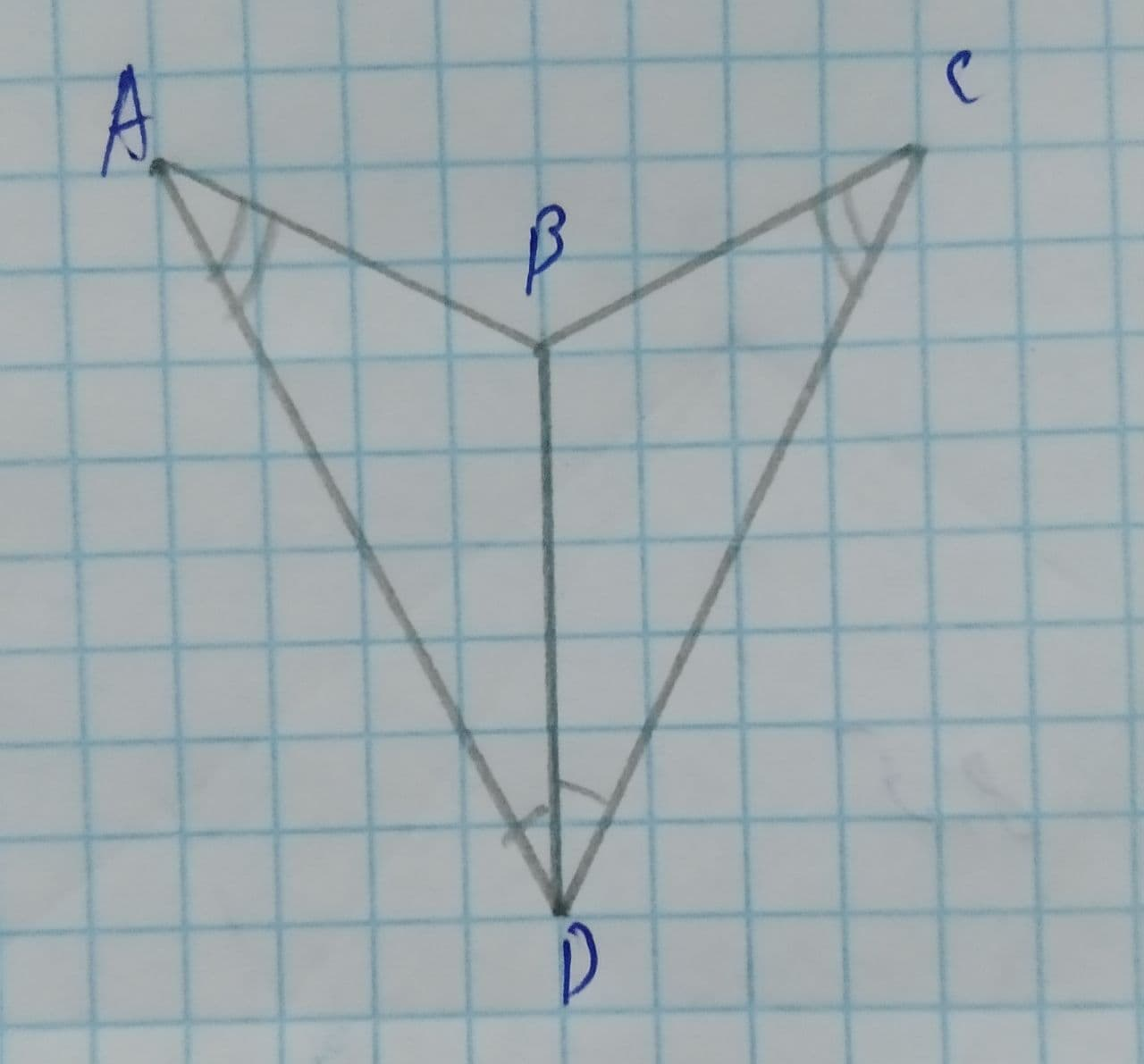# The postulate or theorem that can be used to prove each pair of triangles congruent.Given:The given figure is:Rivka Thorpe 2021-04-02 Answered

The postulate or theorem that can be used to prove each pair of triangles congruent.
Given:
The given figure is:You can still ask an expert for help

• Live experts 24/7
• Questions are typically answered in as fast as 30 minutes
• Personalized clear answers

Solve your problem for the price of one coffee

• Math expert for every subject
• Pay only if we can solve itescumantsu

Calculation:
If the 2 angles and the non-included side of one triangle is congruent to the 2 angles and non-included side of another triangle, then the two triangles are congruent.
Label the diagram given:Angle A is congruent to angle C and angle BDA is congruent to angle CDA.
According to the reflexive property of congruence, line BD is congruent to BD.
Therefore,
$\mathrm{△}BDA\stackrel{\sim }{=}\mathrm{△}BDC$ (AAA Congruence Theorem).

We have step-by-step solutions for your answer!